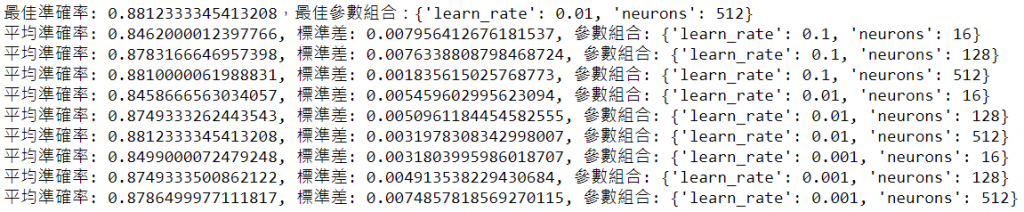#DAY 5
1
AI & Data

## GridSearchCV 函數

GridSearchCV 函數會自動作Cross Validation，並且統計準確率的平均數/標準差，幫我們找出最佳參數組合，我們現在就整合Tensorflow與GridSearchCV，試作一範例說明如下，檔案名稱為04_06_GridSearchCV.ipynb。

1. 首先要使用函數建立模型，並將要測試的參數當作函數的Input。
``````# 建立模型
def create_model(learn_rate=0.01, neurons=128):
model = tf.keras.models.Sequential([
tf.keras.layers.Flatten(input_shape=(28, 28)),
tf.keras.layers.Dense(neurons, activation='relu'),
tf.keras.layers.Dropout(0.2),
tf.keras.layers.Dense(10, activation='softmax')
])
# 設定優化器(optimizer)、損失函數(loss)、效能衡量指標(metrics)的類別
loss='sparse_categorical_crossentropy',
metrics=['accuracy'])
return model
``````
1. 以 KerasClassifier 呼叫建立模型函數。
``````from sklearn.model_selection import GridSearchCV

# 建立模型，並以 KerasClassifier 包起來，提供 GridSearchCV 使用
model = tf.keras.wrappers.scikit_learn.KerasClassifier(build_fn=create_model, epochs=5, verbose=1)
``````
1. 定義參數各種組合，傳入GridSearchCV，呼叫 fit()，即開始評估各個模型準確率。如果設 n_jobs=n，就表示n個組合同步執行，若設為-1，GridSearchCV會使用所有的處理器(processors)同步執行，所以，讀者如果有強大的伺服器，就可以大大的提高生產力了。
``````# 定義參數各種組合
neurons = [16, 128, 512]
learn_rate = [0.1, 0.01, 0.001]
param_grid = dict(learn_rate=learn_rate, neurons=neurons)
grid = GridSearchCV(estimator=model, param_grid=param_grid, n_jobs=1)
# 訓練
grid_result = grid.fit(x_train_norm, y_train)
``````
1. 全部執行完畢，即可取得最佳參數組合及其準確率。
``````# 評估，打分數
print(f"最佳準確率: {grid_result.best_score_}，最佳參數組合：{grid_result.best_params_}")
# 取得 cross validation 的平均準確率及標準差
means = grid_result.cv_results_['mean_test_score']
stds = grid_result.cv_results_['std_test_score']
params = grid_result.cv_results_['params']
for mean, stdev, param in zip(means, stds, params):
print(f"平均準確率: {mean}, 標準差: {stdev}, 參數組合: {param}")
``````